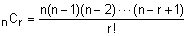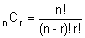## What is combination formula, Mathematics

Assignment Help:

Q. What is Combination Formula?

Ans.

The difference between combinations and permutations is that permutations take ordering into consideration, whereas combinations do not.

A combination is a set of distinct objects without regard to an arrangement or an order.

Combination Formulaor, in factorial notation,#### Ratios, in a veggie mix the ratio of cups of carrots to cups of broccolie i...

in a veggie mix the ratio of cups of carrots to cups of broccolie is 4 to 5 if you made this party mix larger how many cups of carrots would be needed to mix with fo cups of brocco

#### Reflection , How do you reflect about the origin

How do you reflect about the origin

#### Repetition need not be boring-ways to aid learning maths, Repetition Need N...

Repetition Need Not Be Boring :  From an early age on, children engage in and learn from repetitive behaviour, such as dropping and picking up things, opening and closing boxes an

1+1=?

#### Denote the statement in predicate calculus, Denote the subsequent statement...

Denote the subsequent statement in predicate calculus: "Everybody respects all the selfless leaders". Ans: For each X, if every Y that is a person respects X, then X is a selfl

example with solution of quadratic polynomial

#### Theorem to computer the integral, Use green's theorem to computer the integ...

Use green's theorem to computer the integral F . dr where F = ( y^2 + x, y^2 + y) and c is bounded below the curve y= - cos(x),, above by y = sin(x) to the left by x=0 and to the r

#### Area problem, Area Problem Now It is time to start second kind of inte...

Area Problem Now It is time to start second kind of integral: Definite Integrals.  The area problem is to definite integrals what tangent & rate of change problems are to d

lecturer notes

#### Sine series, sum of sine series

sum of sine series